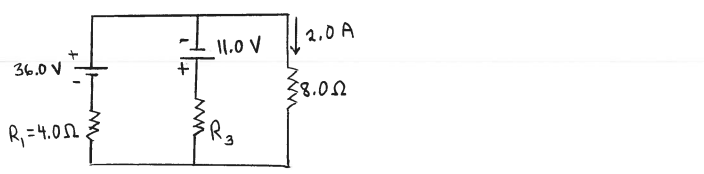# Problem: Consider the circuit shown in the sketch. The current in the 8.0 Ω resistor is 2.0 A, in the direction shown. What is the resistance of the resistor R3?

###### FREE Expert Solution
88% (352 ratings)
###### Problem Details

Consider the circuit shown in the sketch. The current in the 8.0 Ω resistor is 2.0 A, in the direction shown.

What is the resistance of the resistor R3?Frequently Asked Questions

What scientific concept do you need to know in order to solve this problem?

Our tutors have indicated that to solve this problem you will need to apply the Kirchhoff's Loop Rule concept. You can view video lessons to learn Kirchhoff's Loop Rule. Or if you need more Kirchhoff's Loop Rule practice, you can also practice Kirchhoff's Loop Rule practice problems.

How long does this problem take to solve?

Our expert Physics tutor, Juan took 9 minutes and 53 seconds to solve this problem. You can follow their steps in the video explanation above.